# 43 Results

View
Selected filters:
• MI.Math.Content.7.RP.A.2Unrestricted Use
CC BY
Rating

Dan Meyer's Three Act Math task on proportions. Learners work with ratios and proportions, to determine how to fix the incorrectly mixed paint.

Subject:
Mathematics
Material Type:
Activity/Lab
Author:
Dan Meyer
08/06/2020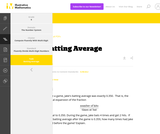Unrestricted Use
CC BY
Rating

This is a task from the Illustrative Mathematics website that is one part of a complete illustration of the standard to which it is aligned. Each task has at least one solution and some commentary that addresses important aspects of the task and its potential use.

Subject:
Numbers and Operations
Material Type:
Activity/Lab
Provider:
Illustrative Mathematics
Provider Set:
Illustrative Mathematics
Author:
Illustrative Mathematics
02/16/2018Unrestricted Use
CC BY
Rating

This is a task from the Illustrative Mathematics website that is one part of a complete illustration of the standard to which it is aligned. Each task has at least one solution and some commentary that addresses important asects of the task and its potential use. Here are the first few lines of the commentary for this task: Chichén Itzá was a Mayan city in what is now Mexico. The picture below shows El Castillo, also known as the pyramid of Kukulcán, which is a pyramid loc...

Subject:
Mathematics
Material Type:
Activity/Lab
Provider:
Illustrative Mathematics
Provider Set:
Illustrative Mathematics
Author:
Illustrative Mathematics
08/07/2013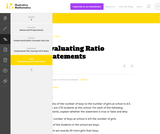Unrestricted Use
CC BY
Rating

This is a task from the Illustrative Mathematics website that is one part of a complete illustration of the standard to which it is aligned. Each task has at least one solution and some commentary that addresses important aspects of the task and its potential use.

Subject:
Ratios and Proportions
Material Type:
Activity/Lab
Provider:
Illustrative Mathematics
Provider Set:
Illustrative Mathematics
Author:
Illustrative Mathematics
02/16/2018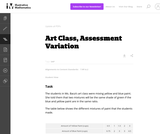Unrestricted Use
CC BY
Rating

This is a task from the Illustrative Mathematics website that is one part of a complete illustration of the standard to which it is aligned. Each task has at least one solution and some commentary that addresses important asects of the task and its potential use. Here are the first few lines of the commentary for this task: The students in Ms. Baca’s art class were mixing yellow and blue paint. She told them that two mixtures will be the same shade of green if the blue and...

Subject:
Mathematics
Material Type:
Activity/Lab
Provider:
Illustrative Mathematics
Provider Set:
Illustrative Mathematics
Author:
Illustrative Mathematics
03/18/2013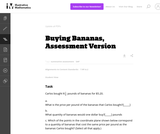Unrestricted Use
CC BY
Rating

This is a task from the Illustrative Mathematics website that is one part of a complete illustration of the standard to which it is aligned. Each task has at least one solution and some commentary that addresses important asects of the task and its potential use. Here are the first few lines of the commentary for this task: Carlos bought $6\frac12$ pounds of bananas for \5.20. What is the price per pound of the bananas that Carlos bought?[_____] What quantity of bananas w... Subject: Mathematics Material Type: Activity/Lab Provider: Illustrative Mathematics Provider Set: Illustrative Mathematics Author: Illustrative Mathematics Date Added: 03/18/2013Unrestricted Use CC BY Rating This is a task from the Illustrative Mathematics website that is one part of a complete illustration of the standard to which it is aligned. Each task has at least one solution and some commentary that addresses important aspects of the task and its potential use. Subject: Ratios and Proportions Material Type: Activity/Lab Provider: Illustrative Mathematics Provider Set: Illustrative Mathematics Author: Illustrative Mathematics Date Added: 02/16/2018Unrestricted Use CC BY Rating This is a task from the Illustrative Mathematics website that is one part of a complete illustration of the standard to which it is aligned. Each task has at least one solution and some commentary that addresses important asects of the task and its potential use. Here are the first few lines of the commentary for this task: Joel and Marisa are running for president at their middle school (grades 6-8). After the votes are in, Joel and Marisa are each convinced that they hav... Subject: Mathematics Material Type: Activity/Lab Provider: Illustrative Mathematics Provider Set: Illustrative Mathematics Author: Illustrative Mathematics Date Added: 08/10/2013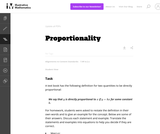Unrestricted Use CC BY Rating This is a task from the Illustrative Mathematics website that is one part of a complete illustration of the standard to which it is aligned. Each task has at least one solution and some commentary that addresses important asects of the task and its potential use. Here are the first few lines of the commentary for this task: A text book has the following definition for two quantities to be directly proportional: We say thaty$is directly proportional to$x$if$y=kx\$ for ...

Subject:
Mathematics
Material Type:
Activity/Lab
Provider:
Illustrative Mathematics
Provider Set:
Illustrative Mathematics
Author:
Illustrative Mathematics
07/16/2013Unrestricted Use
CC BY
Rating

This is a task from the Illustrative Mathematics website that is one part of a complete illustration of the standard to which it is aligned. Each task has at least one solution and some commentary that addresses important asects of the task and its potential use. Here are the first few lines of the commentary for this task: The students in Carli’s class built some solar-powered robots which they raced in the cafeteria of the school. http://www.youtube.com/watch?v=wOI66f4BS...

Subject:
Mathematics
Material Type:
Activity/Lab
Provider:
Illustrative Mathematics
Provider Set:
Illustrative Mathematics
Author:
Illustrative Mathematics
03/18/2013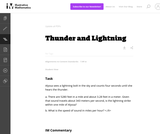Unrestricted Use
CC BY
Rating

This is a task from the Illustrative Mathematics website that is one part of a complete illustration of the standard to which it is aligned. Each task has at least one solution and some commentary that addresses important asects of the task and its potential use. Here are the first few lines of the commentary for this task: Alyssa sees a lightning bolt in the sky and counts four seconds until she hears the thunder. There are 5280 feet in a mile and about 3.28 feet in a met...

Subject:
Mathematics
Material Type:
Activity/Lab
Provider:
Illustrative Mathematics
Provider Set:
Illustrative Mathematics
Author:
Illustrative Mathematics
08/07/2013Unrestricted Use
CC BY
Rating

This is a task from the Illustrative Mathematics website that is one part of a complete illustration of the standard to which it is aligned. Each task has at least one solution and some commentary that addresses important asects of the task and its potential use. Here are the first few lines of the commentary for this task: Julianna participated in a walk-a-thon to raise money for cancer research. She recorded the total distance she walked at several different points in ti...

Subject:
Mathematics
Material Type:
Activity/Lab
Provider:
Illustrative Mathematics
Provider Set:
Illustrative Mathematics
Author:
Illustrative Mathematics
07/07/2013Unrestricted Use
CC BY
Rating

This course is an arithmetic course intended for college students, covering whole numbers, fractions, decimals, percents, ratios and proportions, geometry, measurement, statistics, and integers using an integrated geometry and statistics approach. The course uses the late integers modelintegers are only introduced at the end of the course.

Subject:
Mathematics
Material Type:
Full Course
Textbook
Provider:
Lumen Learning
Provider Set:
Candela Courseware
Author:
David Lippman
02/16/2018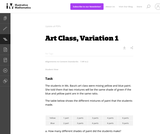Unrestricted Use
CC BY
Rating

This this task about mixing paint requires students to graph ratios on a coordinate plane. It is a standard language in ratio problem.

Subject:
Mathematics
Material Type:
Activity/Lab
Provider:
Illustrative Mathematics
Provider Set:
Illustrative Mathematics
Author:
Illustrative Mathematics
05/01/2012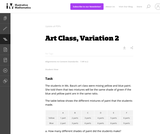Unrestricted Use
CC BY
Rating

This this task about mixing paint requires students to graph ratios on a coordinate plane. It is a standard language in ratio problem.

Subject:
Mathematics
Material Type:
Activity/Lab
Provider:
Illustrative Mathematics
Provider Set:
Illustrative Mathematics
Author:
Illustrative Mathematics
05/01/2012Conditional Remix & Share Permitted
CC BY-NC-SA
Rating

This Cyberchase video segment features Bianca, who must figure out the fastest route to a movie premiere.

Subject:
Mathematics
Physics
Material Type:
Lecture
08/04/2020Conditional Remix & Share Permitted
CC BY-NC-SA
Rating

The eye’s retina receives and reacts to incoming light and sends signals to the brain, allowing you to see. One part of the retina, however, doesn't give you visual information—this is your eye’s “blind spot.”

Subject:
Anatomy/Physiology
Material Type:
Activity/Lab
Provider:
Exploratorium
Provider Set:
Science Snacks
09/04/2019Unrestricted Use
CC BY
Rating

This is a task where it would be appropriate for students to use technology such as a graphing calculator or GeoGebra, making it a good candidate for students to engage in Standard for Mathematical Practice 5 Use appropriate tools strategically.

Subject:
Mathematics
Material Type:
Activity/Lab
Provider:
Illustrative Mathematics
Provider Set:
Illustrative Mathematics
Author:
Illustrative Mathematics
05/01/2012Conditional Remix & Share Permitted
CC BY-NC-SA
Rating

Includes chapters on: Basics of Geometry, Reasoning and Proof, Parallel and Perpendicular Lines, Triangles and Congruence, Relationships with Triangles, Polygons and Quadrilaterals, Similarity, Right Triangle Trigonometry, Circles, Perimeter and Area, Surface Area and Volume, Rigid Transformations.

Subject:
Mathematics
Geometry
Material Type:
Textbook
Provider:
CK-12 Foundation
10/15/2014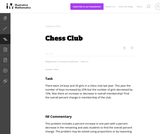Unrestricted Use
CC BY
Rating

This problem includes a percent increase in one part with a percent decrease in the remaining and asks students to find the overall percent change. The problem may be solved using proportions or by reasoning through the computations or writing a set of equations.

Subject:
Mathematics
Material Type:
Activity/Lab
Provider:
Illustrative Mathematics
Provider Set:
Illustrative Mathematics
Author:
Illustrative Mathematics